## Minimization of DFA-

 The process of reducing a given DFA to its minimal form is called as minimization of DFA.

• It contains the minimum number of states.
• The DFA in its minimal form is called as a Minimal DFA.

## How To Minimize DFA?

The two popular methods for minimizing a DFA are-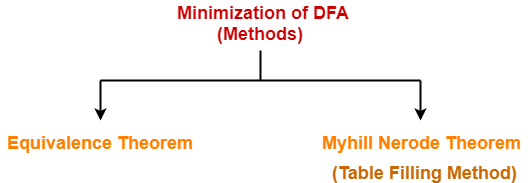In this article, we will discuss Minimization of DFA Using Equivalence Theorem.

## Minimization of DFA Using Equivalence Theorem-

### Step-01:

• Eliminate all the dead states and inaccessible states from the given DFA (if any).

All those non-final states which transit to itself for all input symbols in ∑ are called as dead states.

### Inaccessible State

All those states which can never be reached from the initial state are called as inaccessible states.

### Step-02:

• Draw a state transition table for the given DFA.
• Transition table shows the transition of all states on all input symbols in Σ.

### Step-03:

Now, start applying equivalence theorem.

• Take a counter variable k and initialize it with value 0.
• Divide Q (set of states) into two sets such that one set contains all the non-final states and other set contains all the final states.
• This partition is called P0.

### Step-04:

• Increment k by 1.
• Find Pk by partitioning the different sets of Pk-1 .
• In each set of Pk-1 , consider all the possible pair of states within each set and if the two states are distinguishable, partition the set into different sets in Pk.

 Two states q1 and q2 are distinguishable in partition Pk for any input symbol ‘a’,if δ (q1, a) and δ (q2, a) are in different sets in partition Pk-1.

### Step-05:

• Repeat step-04 until no change in partition occurs.
• In other words, when you find Pk = Pk-1, stop.

### Step-06:

• All those states which belong to the same set are equivalent.
• The equivalent states are merged to form a single state in the minimal DFA.

 Number of states in Minimal DFA= Number of sets in Pk

## Problem-01:

Minimize the given DFA-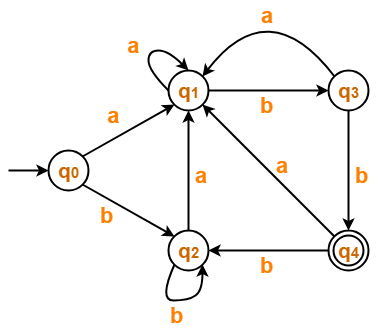## Solution-

### Step-01:

The given DFA contains no dead states and inaccessible states.

### Step-02:

Draw a state transition table-

 a b →q0 q1 q2 q1 q1 q3 q2 q1 q2 q3 q1 *q4 *q4 q1 q2

### Step-03:

Now using Equivalence Theorem, we have-

P0 = { q0 , q1 , q2 , q3 } { q4 }

P1 = { q0 , q1 , q2 } { q3 } { q4 }

P2 = { q0 , q2 } { q1 } { q3 } { q4 }

P3 = { q0 , q2 } { q1 } { q3 } { q4 }

Since P3 = P2, so we stop.

From P3, we infer that states q0 and q2 are equivalent and can be merged together.

So, Our minimal DFA is-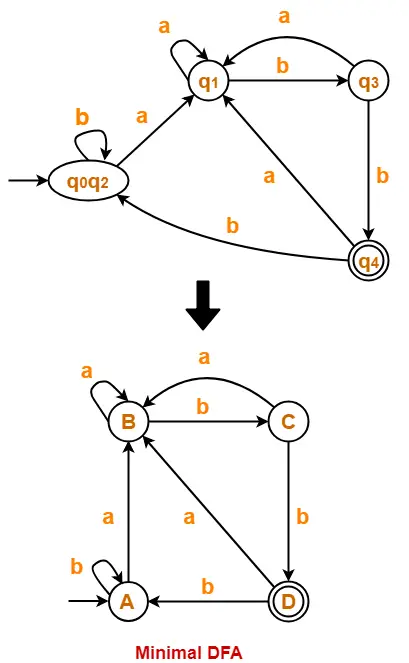## Problem-02:

Minimize the given DFA-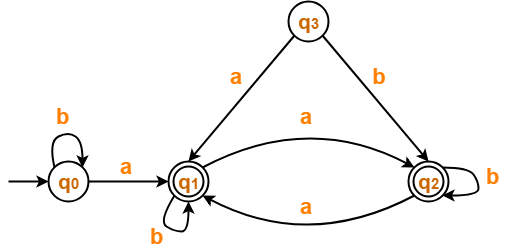## Solution-

### Step-01:

• State q3 is inaccessible from the initial state.
• So, we eliminate it and its associated edges from the DFA.

The resulting DFA is-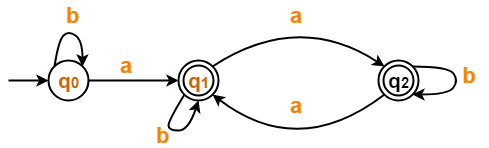### Step-02:

Draw a state transition table-

 a b →q0 *q1 q0 *q1 *q2 *q1 *q2 *q1 *q2

### Step-03:

Now using Equivalence Theorem, we have-

P0 = { q0 } { q1 , q2 }

P1 = { q0 } { q1 , q2 }

Since P1 = P0, so we stop.

From P1, we infer that states q1 and q2 are equivalent and can be merged together.

So, Our minimal DFA is-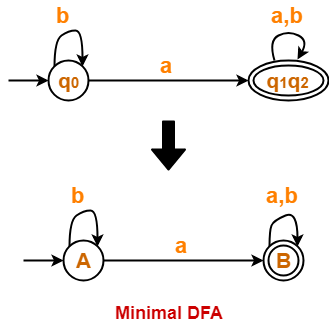## Problem-03:

Minimize the given DFA-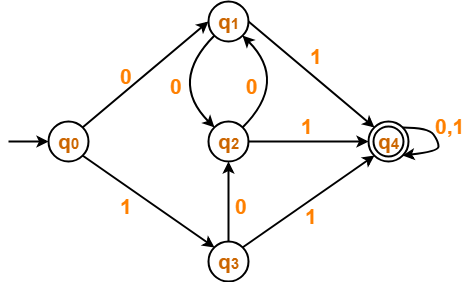## Solution-

### Step-01:

The given DFA contains no dead states and inaccessible states.

### Step-02:

Draw a state transition table-

 0 1 →q0 q1 q3 q1 q2 *q4 q2 q1 *q4 q3 q2 *q4 *q4 *q4 *q4

### Step-03:

Now using Equivalence Theorem, we have-

P0 = { q0 , q1 , q2 , q3 } { q4 }

P1 = { q0 } { q1 , q2 , q3 } { q4 }

P2 = { q0 } { q1 , q2 , q3 } { q4 }

Since P2 = P1, so we stop.

From P2, we infer that states q1 , q2 and q3 are equivalent and can be merged together.

So, Our minimal DFA is-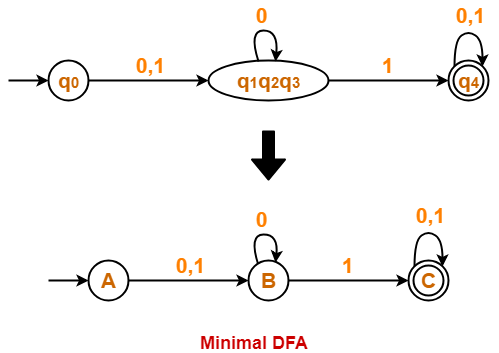## Problem-04:

Minimize the given DFA-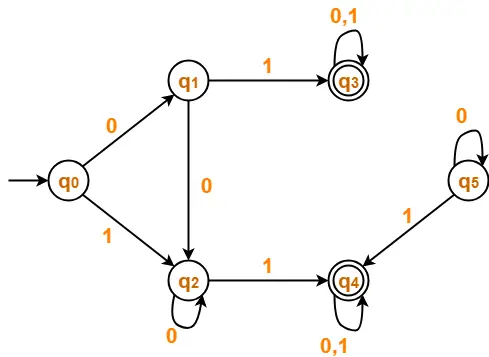## Solution-

### Step-01:

• State q5 is inaccessible from the initial state.
• So, we eliminate it and its associated edges from the DFA.

The resulting DFA is-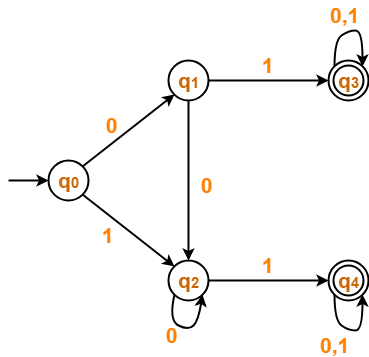### Step-02:

Draw a state transition table-

 0 1 →q0 q1 q2 q1 q2 *q3 q2 q2 *q4 *q3 *q3 *q3 *q4 *q4 *q4

### Step-03:

Now using Equivalence Theorem, we have-

P0 = { q0 , q1 , q2 } { q3 , q4 }

P1 = { q0 } { q1 , q2 } { q3 , q4 }

P2 = { q0 } { q1 , q2 } { q3 , q4 }

Since P2 = P1, so we stop.

From P2, we infer-

• States q1 and q2 are equivalent and can be merged together.
• States q3 and q4 are equivalent and can be merged together.

So, Our minimal DFA is-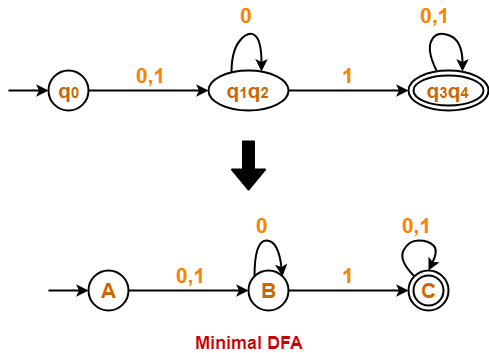To gain better understanding about Minimization of DFA,

Watch this Video Lecture

Next Article- Converting DFA to Regular Expression

Get more notes and other study material of Theory of Automata and Computation.

Watch video lectures by visiting our YouTube channel LearnVidFun.